# Digisim® Tutorials

#### 2. Entering a Mechanism and Parameters

DigiSim® is based on the Fast Implicit Finite Difference method developed by Manfred Rudolph, which is arguably the most general and most efficient method currently available for digital simulation. In addition to these attributes, DigiSim is easy to use, and requires little mathematical sophistication or computer expertise on the part of the user. In this note and following notes, we will briefly examine some of the applications of DigiSim (for more detail, see the review by Rudolph and Feldberg (1)).

DigiSim can simulate the cyclic voltammogram for any solution redox mechanism that can be expressed in terms of single or multiple-electron transfer reactions and first- or second-order chemical reactions. To enter a new mechanism, select New from the File menu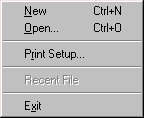then select CV-Properties from the Edit menu.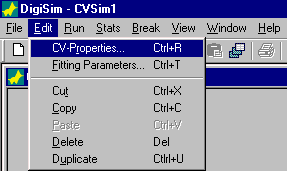There are 4 parts to the CV-Properties dialog box.

#### Mechanism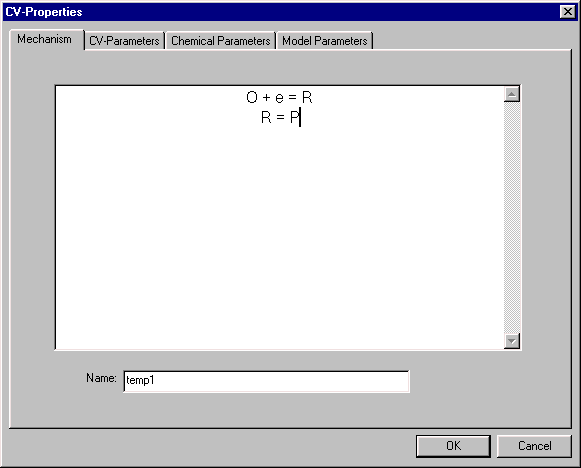This input screen is used to enter a mechanism. In this example, an EC mechanism (electron transfer followed by a chemical reaction) has been entered.

#### CV-Parameters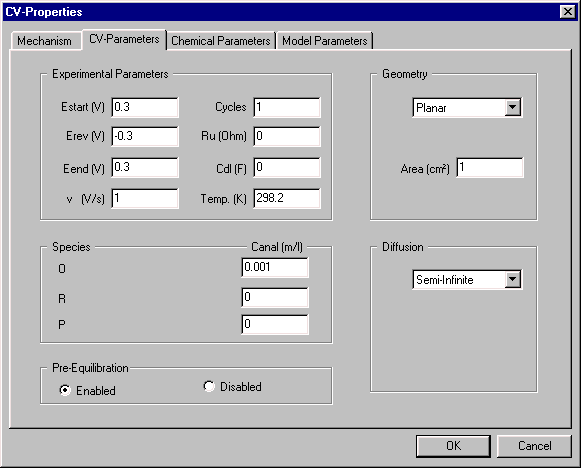This dialog box is used to defined the parameters of the CV experiment, which are as follows:

• the potential range of the experiment (Estart, Erev, and Eend)
• the scan rate (v)
• the number of potential Cycles
• solution resistance (Ru)and double layer capacitance (Cdl)
• the electrode Geometry (e.g., planar, spherical) and surface Area
• type of Diffusion (semi-infinite, finite, or hydrodynamic)
• concentrations of the species involved in the redox mechanisms (Canal)

#### Chemical ParametersThis dialog box is used to enter thermodynamic and kinetic parameters for the reactions involved in the mechanism. The following must be defined for each (Heterogeneous) electron transfer reaction:

• the redox potential (Eo)
• the mathematical model for the kinetics (Butler-Volmer or Marcus (2))
• the transfer coefficient (a/l)
• the electron transfer rate constant (ks)

Three parameters are required for each chemical (Homogeneous) reaction; the equilibrium constant (Keq), and the rates of the forward and reverse reaction (kf and kb). There are two important points to note about these three parameters:

• Only two parameters Keq and kf can be defined by the user, since kb = kf/Keq.
• DigiSim conforms to the principle of microscopic reversibility, which requires a reaction to be able to proceed in both directions; that is, there cannot be a completely irreversible reaction. However, a reaction for which kb is very small is effectively equivalent to an irreversible reaction.

The Species Parameters are also entered in this dialog box. These are the diffusion coefficients (D) and concentrations of all the species involved in the redox mechanism. Two concentrations are shown. The analytical concentrations (Canal) are entered by the user (either in this or the CV-Parameters dialog box), and correspond to the concentrations that are added to the solution. The initial concentrations (Cinit) are the equilibrium concentrations at the electrode surface, and are determined by Estart, all Eo values, all Keq values, and all Canal values. It is the Cinit values rather than the Canal values that are used in the simulation. The calculation of the Cinit values can be switched off by disabling the Pre-Equilibration in the CV-Parameters dialog box (if the calculation of Cinit is disabled, the Canal values are used in the simulation).

#### Model Parameters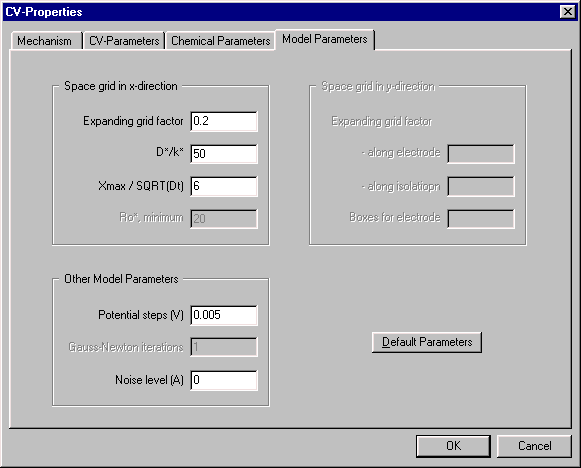The parameters in this dialog box are related to the calculation. The most important parameters are the Expanding grid factor and the Potential steps, which specify the resolution of the space and time grids, respectively, that are used in the simulation. Entering lower values for either parameter will increase the resolution of the grid, which may increase the accuracy of the simulation (however, there is a point beyond which further increases in resolution will have no effect). Increasing the grid resolution will also increase the time required for the calculation, but this is generally no longer an issue with the speed of PCs now available. There are two occasions when decreasing the Expanding grid factor is useful, and these are discussed in later notes (CV - the MovieTM and How Do You Know It's Right?)

#### Reference

1. M. Rudolph, D.P. Reddy and S.W. Feldberg, Anal. Chem. 66 (1994) 589A.
2. Butler-Volmer is the default kinetic model, and is the more commonly used. Marcusian kinetics is used only for a few, well-defined situations.

DigiSim is a registered trademark and CV - the Movie is a trademark of Bioanalytical System, Inc.